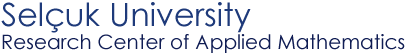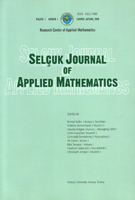Selçuk Journal of Applied Mathematics www.selcuk.edu.trSelçuk Journal of
Applied Mathematics

Winter-Spring, 2002
Volume  3
Number 1

Research Center of
Applied Mathematics

 SJAM Winter-Spring 2002, Volume 3 - Number 1 Resolvent of matrix polynomials, pseudospectra and inversion problems Jan Kozánek* Institute of Thermomechanics, Academy of Sciences of the Czech Republic,  Dolejskova 5, 182 00 Prague 8, Czech Republic. email: kozanek@it.cas.cz Received: March 22, 2002 Summary This paper deals with the mathematical model of a dynamic systems written by the n ordinary differential equations of order m with constant coefficients. The formulas for the resolvent of matrix polynomial (l-matrix problem) and for "derivative resolvents", as well theirs derivations with respect to l are given with help of less commonly used linearization form. The extension of Pseudospectra definition for above matrix polynomial problem is proposed. The inversion problem, formulated here for general complex and real coefficient matrices is important in numerical simulation. As a motivation of the study of an evolutive dynamic system depending on a one parameter, the famous Lancaster's example, where m=2 and n=4, is given. Key words dynamic systems, regular matrix polynomial problem, linearization, eigenvalue, eigenvector, latent roots, latent vector, generalized latent vector, Jordan canonical form, resolvent, state space, component matrices, pseudospectra, inversion problem Mathematics Subject Classification (1991): 34A30, 47A10, 15A18, 15A22, 34A55 * This research was supported by the Grant Agency of the Czech Republic (No 101/00/1471). Article in PS format (314 Kb)
 The article is available to read in  Ghostview format
 [Home] [contact]